HTML 5 Physics Games
The games listed below are programs that I wrote for my students to use to help them master a specific skill they need to use in my class. These programs were written to work on computers, tablets, phones and other handheld devices with HTML 5 capable browsers. All functionality for these programs comes from using the mouse or the touch screen an no keyboard usage is required.Timing Challenge Students must show that they can successfully time an event using a hand held stopwatch. Possible Student Directions YouTube of how to use the program.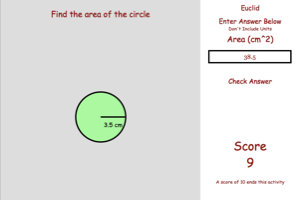Geometry Review Self Test Students must show that they can calculate basic distances, areas and volumes for simple geometric shapes. Possible Student Directions YouTube of how to use the program.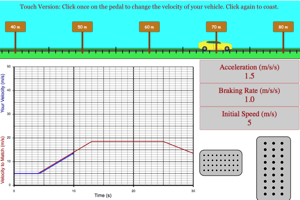Velocity Graph Matching Challenge Challenges students recreate a velocity vs time graph by setting the initial speed, the positive acceleration, and the braking rate. Students then use the gas and brake on the car to match the graph. Possible Student Directions YouTube of how to use the program.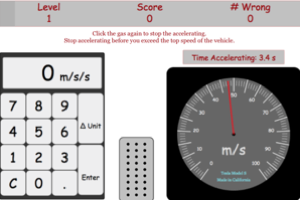Acceleration Challenge Challenges students to get as vehicle accelerations as possible in a 3 minute time frame. Students must push on the gas to accelerate their vehicle and then use the change in velocity and the change in time to get the acceleration of the vehicle. Possible Student Directions YouTube of how to use the program.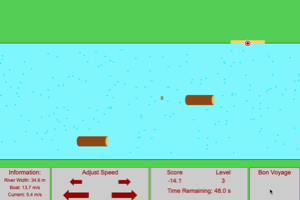Normal River Crossing Challenge Challenges students to get as many boats to their targets on the opposite side of a river as possible. In this version the boat will be launched perpendicular to the river and students must set the velocity to get the boat to the target on the other side. Possible Student Directions YouTube of how to use the program.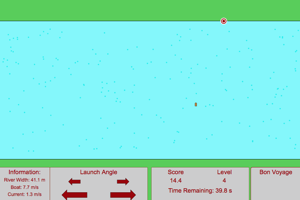River Crossing Challenge Challenges students to get as many boats to their targets on the opposite side of a river as possible. Gives students a hands on experience with 2d relative velocity. Possible Student Directions YouTube of how to use the program.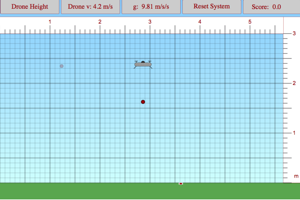Drone Delivery Challenge Challenges students to land as many objects as close to the target as possible. More points will be earned if the height is high and the speed is fast. Possible Student Directions YouTube of how to use the program.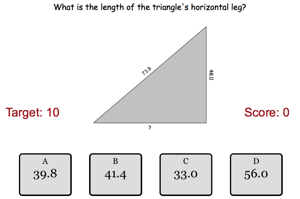Triangle Math Challenge Challenges students figure out ten unknown values for right triangles without getting any problems wrong. Possible Student Directions YouTube of how to use the program.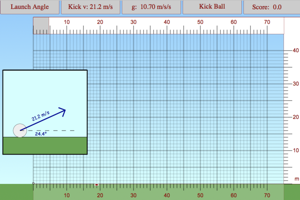Soccer Kick Challenge Challenges students to hit as many targets as possible by adjusting the angle of the kick until the angle will create the perfect range for landing on the target. Possible Student Directions YouTube of how to use the program.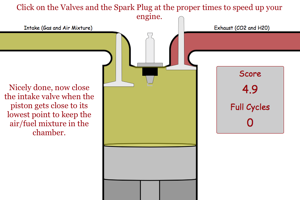Internal Combustion Engine Challenge Checks that students understand the steps involved in an internal combustion engine. Possible Student Directions YouTube of how to use the program.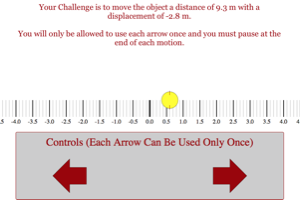Distance and Displacement Challenge Checks that students are able to apply the ideas of distance and displacement to move an object using the proper sequence of steps. Possible Student Directions YouTube of how to use the program.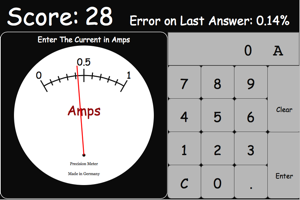Read the Meter Challenge Checks that students are able to read an analog current meter. Possible Student Directions YouTube of how to use the program.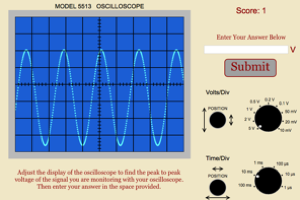Read the Oscilloscope Challenge Checks that students are able to read an oscilloscope. Students must achieve a score or 10 or more. At the end students should be able to get frequency, period, voltage and peak to peak voltage. Possible Student Directions YouTube of how to use the program.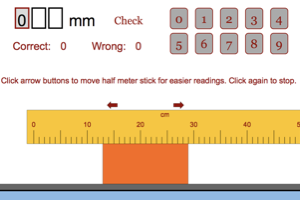Read the Ruler Challenge Checks that students are able to measure the lengths of a few different objects. Possible Student Directions YouTube of how to use the program.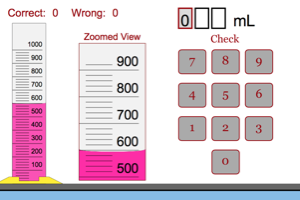Graduated Cylinder Challenge Checks that students are able to read a few different sized graduated cylinders. Possible Student Directions YouTube of how to use the program.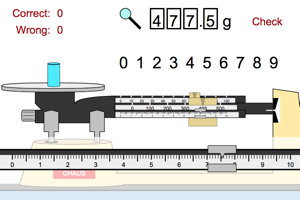Triple-Beam Balance Challenge (0.1 g) Challenge Checks that students are able to read a triple-beam balance to the nearest 0.1 g. Possible Student Directions YouTube of how to use the program.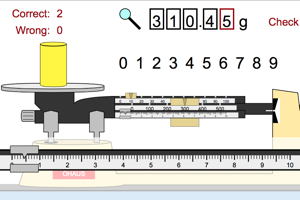Triple-Beam Balance Challenge (0.01 g) Challenge Checks that students are able to read a triple-beam balance to the nearest 0.01 g. Possible Student Directions YouTube of how to use the program.Read the Thermometer Challenge Checks that students are able to read thermometers. Possible Student Directions YouTube of how to use the program.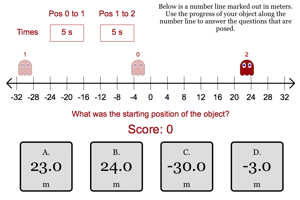Motion Terminology Challenge This challenge is designed to make sure students can apply the motion terms to randomly generated series of motions. Possible Student Directions YouTube of how to use the program.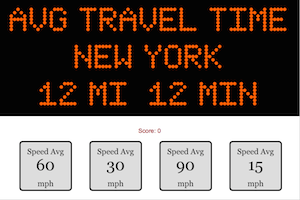Speed in Traffic Game This game is designed to help students use mental math to find the average speed of traffic. Possible Student Directions YouTube of how to use the program.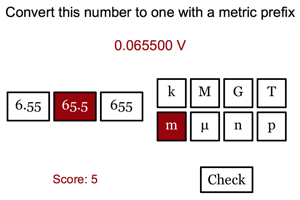Metric Prefix Game This game is designed to help students learn the metric prefixes and how to convert large and small numbers into numbers written with a metric prefix. YouTube of how to use the program.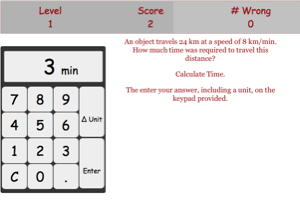Distance and Velocity Game This game is designed to help students mentally figure out distances, speeds or time for objects moving at constant speed. YouTube of how to use the program.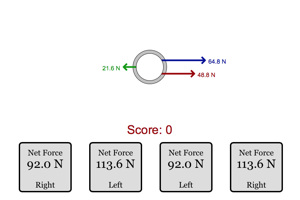Linear Net Force Game This game is intended to help students master the idea of net force for parallel forces. Students will have two minutes to figure out as many net forces as possible. YouTube of how to use the program.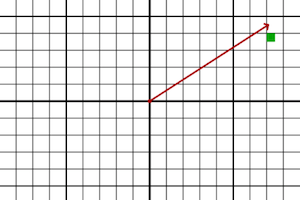One Step Vector Treasure Hunt Game This game is intended to help students break a vector into components. They will be given a vector and then have click to see if they hit the right spot. Students need a score of 5 to successfully complete this activity. YouTube of how to use the program.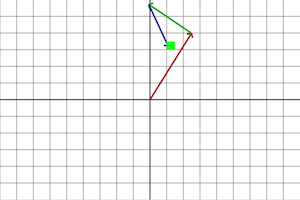Vector Treasure Hunt Game This game is intended to help students get the idea of vector addition. Students will be given a randomly generated series of three vectors. They must follow the vectors to the end and then click to see if they hit the right spot. YouTube of how to use the program.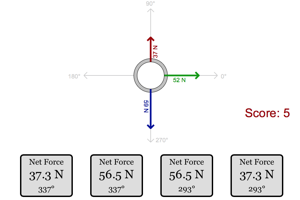Non-Linear Net Force Game This game is intended to help students master the idea of net force for non-parallel forces. Students will have three minutes to figure out as many net forces as possible. YouTube of how to use the program.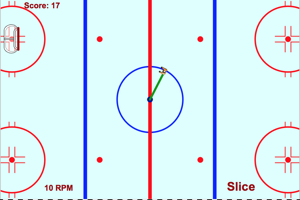Slice the String This game is intended to give students an intuitive feel for the direction the velocity for an object in circular motion. The objects is to score as many points in two minutes as possible. You earn more points the closer you are to the center of the goal and the higher the RPM when you score. The RPM increase by 5 after each goal. Each time you miss the net, you lose points equivalent to 2*#RPM and your RPM will drop by 1. YouTube of how to use the program.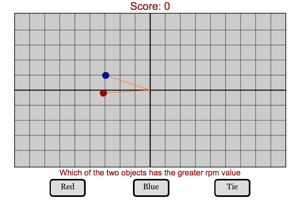Circular Motion Self Test This game is intended to help students master the basic terms for circular motion. Students will have two minutes to correctly answer as many questions as possible. YouTube of how to use the program.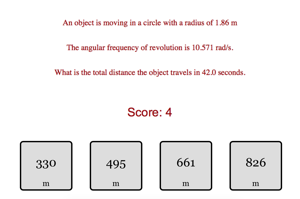Quick Circular Motion Calculation Challenge This challenge is designed to help students move back and forth from different circular motion ideas. These ideas include frequency, rpm, period, angular speed, linear speed, total distance and total radians. YouTube of how to use the program.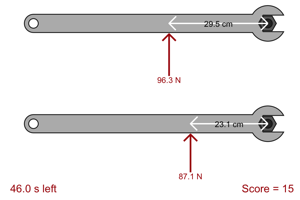Torque Game This game is intended to help students mentally judge the amount of torque based on distance and force. They will be presented with two different wrenches and they must decide which wrench has the greater torque on it. YouTube of how to use the program.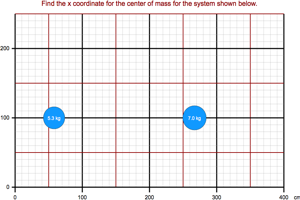Center of Mass Level 1 Find the center of mass of a one dimensional system of 2 particles. Possible Student Directions YouTube of how to use the program.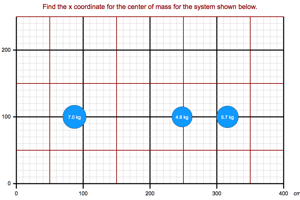Center of Mass Level 2 Find the center of mass of a one dimensional system of 3 particles. Possible Student Directions YouTube of how to use the program.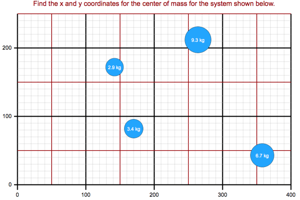Center of Mass Level 3 Find the center of mass of a two dimensional system of 4 particles. Possible Student Directions YouTube of how to use the program.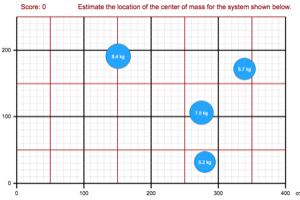Center of Mass Estimated Estimate the location of the center of mass of systems of particles. You will have one minute to estimate as many centers of mass as possible. Possible Student Directions YouTube of how to use the program.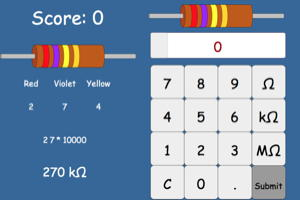Read the Resistor This game will help students learn their color codes for resistors. Students will be given a resistor and they have to determine the resistance of the resistor. Students will have three minutes to get as many correct as possible. YouTube of how to use the program.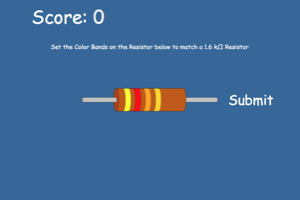Create the Resistor This game will help students learn their color codes for resistors. Students will be given a resistance and they have to determine which combination of colors will match that resistance. Students will have three minutes to get as many correct as possible. YouTube of how to use the program.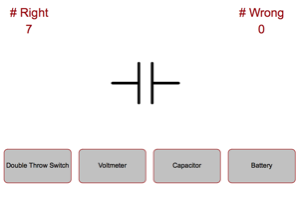Schematic Symbol Identification This game is designed to help students learn the names of some of the schematic symbols they will encounter in electric circuit diagrams. YouTube of how to use the program.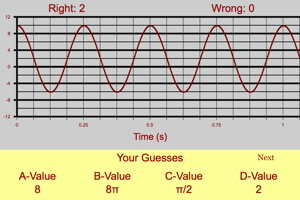Equations of Sine Curves This game will help students determine the coefficients of sine curves. Students must determine the A, B, C and D values for a randomly generated sine curve. YouTube of how to use the program.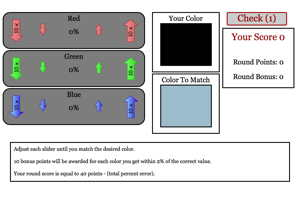Color Matching Game This game has been built to show students that they can make any color they want by mixing different amounts of red, blue and green. YouTube of how to use the program.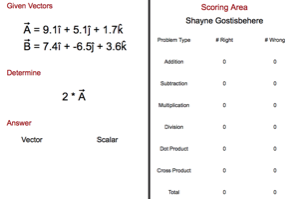3d Vector Math Challenge This challenge is designed to have students practice their three dimensional vector math skills. Students will be asked to add and subtract vectors, multiply and diving vectors by a number, and use the vector dot and cross products. Possible Student Directions YouTube of how to use the program.